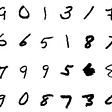# Image Classification on Grape Leaves Disease using Deep Learning

`dataset_name = "esca_dataset"# Url to repo (repo temporary saved in Google Drive but intended to Mendeley repo)dataset_url =  "https://drive.google.com/file/d/1qO997Wy5drvRpVbAOCL20w82FEGiDpmV/view?usp=sharing"   # Google Drive -> to change with Mendely Link# Trick to use wget with gDrive: use 'https://docs.google.com/uc?export=download&id=FILEID'# where FILEID is extracted from the virtual link provided from Google drivedataset_url4wget = "https://docs.google.com/uc?export=download&id=1qO997Wy5drvRpVbAOCL20w82FEGiDpmV"# Download the archive directly from url!wget -r --no-check-certificate "\$dataset_url4wget" -O \$dataset_name".zip"!ls# Unzip data!unzip  \$dataset_name".zip"!ls`
`# The new dataset 'augmented_esca_dataset' will be created.# This dataset contains the augmented images create by the ImageGenerator class and the orginal images,# in order to obtain an expanded version of the orginal dataset ready-to-usefrom keras.preprocessing.image import ImageDataGenerator, array_to_img, img_to_array, load_imgimport tensorflow as tfimport osfrom numpy import expand_dimsimport cv2import matplotlib.pyplot as pltfrom pathlib import Pathdef blur(img):return (cv2.blur(img,(30,30)))def horizontal_flip(img):return (tf.image.flip_left_right(img))def vertical_flip(img):return (tf.image.flip_up_down(img))def contrast(img):return (tf.image.adjust_contrast(img, 0.5))def saturation(img):return (tf.image.adjust_saturation(img, 3))def hue(img):return (tf.image.adjust_hue(img, 0.1))def gamma(img):return (tf.image.adjust_gamma(img, 2))new_dataset = 'augmented_esca_dataset'classes = ['esca', 'healthy']for class_tag in classes:input_path = '/content/' + dataset_name + '/' + class_tag + '/'output_path = '/content/' + dataset_name + '/' + new_dataset + '/' + class_tag + '/'print(input_path)print(output_path)# TMP!rm -rf \$output_path# END TMPtry:if not os.path.exists(output_path):os.makedirs(output_path)except OSError:print ("Creation of the directory %s failed\n\n" % output_path)else:print ("Successfully created the directory %s\n\n" % output_path)for filename in os.listdir(input_path):if filename.endswith(".jpg"):# Copy the original image in the new datasetoriginal_file_path = input_path + filenameoriginal_newname_file_path = output_path + Path(filename).stem + "_original.jpg"%cp \$original_file_path \$original_newname_file_path# Initialising the ImageDataGenerator class.# We will pass in the augmentation parameters in the constructor.for transformation in transformation_array:if transformation == "horizontalFlip":#datagen = ImageDataGenerator(horizontal_flip = True)                 # for random flipdatagen = ImageDataGenerator(preprocessing_function=horizontal_flip)  # all imgs flippedelif transformation == "verticalFlip":#datagen = ImageDataGenerator(vertical_flip = True)                   # for random flipdatagen = ImageDataGenerator(preprocessing_function=vertical_flip)    # all imgs flippedelif transformation == "rotation":datagen = ImageDataGenerator(rotation_range = 40, fill_mode='nearest')elif transformation == "widthShift":datagen = ImageDataGenerator(width_shift_range = 0.2, fill_mode='nearest')elif transformation == "heightShift":datagen = ImageDataGenerator(height_shift_range = 0.2, fill_mode='nearest')elif transformation == "shearRange":datagen = ImageDataGenerator(shear_range = 0.2)elif transformation == "zoom":datagen = ImageDataGenerator(zoom_range = [0.5, 1.0])elif transformation == "blur":datagen = ImageDataGenerator(preprocessing_function=blur)elif transformation == "brightness":#Values less than 1.0 darken the image, e.g. [0.5, 1.0],#whereas values larger than 1.0 brighten the image, e.g. [1.0, 1.5],#where 1.0 has no effect on brightness.datagen = ImageDataGenerator(brightness_range = [1.1, 1.5])elif transformation == "contrast":datagen = ImageDataGenerator(preprocessing_function=contrast)elif transformation == "saturation":datagen = ImageDataGenerator(preprocessing_function=saturation)elif transformation == "hue":datagen = ImageDataGenerator(preprocessing_function=hue)elif transformation == "gamma":datagen = ImageDataGenerator(preprocessing_function=gamma)# Loading a sample imageimg = load_img(input_path + filename)# Converting the input sample image to an arraydata = img_to_array(img)# Reshaping the input image expand dimension to one samplesamples = expand_dims(data, 0)# Plot original imageprint("Original image:")print(filename)if enable_show:plt.imshow(img)plt.show()print("\n\n")# Generating and saving n_augmented_images augmented samplesprint("Apply " + transformation + ".")# prepare iteratorit = datagen.flow(samples, batch_size = 1,save_to_dir = output_path,save_prefix = Path(filename).stem + "_" + transformation,save_format ='jpg')batch = it.next()# Plot trasnformed imageimage = batch.astype('uint8')if enable_show:print("Transformed image:")plt.imshow(image)plt.show()print("\n\n")print("Done!\n\n")`

## Import All Important Dependencies

`import tensorflow as tffrom tensorflow import kerasfrom tensorflow.keras.models import load_modelfrom tensorflow.keras.models import Sequentialfrom tensorflow.keras.layers import Conv2D, MaxPooling2Dfrom tensorflow.keras.layers import Activation, Dropout, Flatten, Densefrom tensorflow.keras.preprocessing import image_dataset_from_directoryimport numpy as npimport matplotlib.pyplot as pltimport osimport time`

## Extract Our Dataset Information

`data_dir = pathlib.Path(dir_original)#Import dataset directoryset_samples = ['train', 'validation', 'test']print("set_samples: ", set_samples, "\n")CLASS_NAMES = np.array([item.name for item in sorted(data_dir.glob('*'))])print("class: ", CLASS_NAMES, "\n")N_IMAGES = np.array([len(list(data_dir.glob(item.name+'/*.jpg'))) for item in sorted(data_dir.glob('*'))])      # number of images for classprint("number of images for class: ", N_IMAGES, "\n")N_samples = np.array([(int(np.around(n*60/100)), int(np.around(n*15/100)), int(np.around(n*25/100))) for n in N_IMAGES])  # number of images for set (train,validation,test)print("split of dataset: \n ", N_samples, "\n")`

## Model Architecture

`model = Sequential()model.add(Conv2D(32, (3, 3), padding='same', input_shape=input_shape))model.add(Activation('relu'))model.add(MaxPooling2D(pool_size=(2, 2)))model.add(Conv2D(32, (3, 3), padding='same'))model.add(Activation('relu'))model.add(MaxPooling2D(pool_size=(2, 2)))model.add(Conv2D(64, (3, 3), padding='same'))model.add(Activation('relu'))model.add(MaxPooling2D(pool_size=(2, 2)))model.add(Conv2D(64, (3, 3), padding='same'))model.add(Activation('relu'))model.add(MaxPooling2D(pool_size=(2, 2)))model.add(Conv2D(32, (3, 3), padding='same'))model.add(Activation('relu'))model.add(MaxPooling2D(pool_size=(2, 2)))model.add(Flatten())model.add(Dense(64))model.add(Activation('relu'))model.add(Dropout(0.5))model.add(Dense(2)) #because we have 2 classmodel.add(Activation('softmax'))model.summary()`

## Model Compilation & Training

`#---compilation----#model.compile(loss='categorical_crossentropy',optimizer=keras.optimizers.Adadelta(learning_rate=1, name='Adadelta'),metrics['accuracy'])#----training----#with tf.device('/device:GPU:0'):history = model.fit(train_dataset,epochs=epochs,validation_data=validation_dataset)model.save('your directory') #save your model in any file path you want`

## InceptionV3

`import kerasfrom keras.applications.inception_v3 import InceptionV3from keras.models import Model,load_modelconv_base =  InceptionV3(weights='imagenet',include_top=False,input_shape=(300, 300, 3))output = conv_base.layers[-1].outputoutput = keras.layers.Flatten()(output)model_tl = Model(conv_base.input, output)model_tl.trainable = Falsefor layer in model_tl.layers:layer.trainable = Falselayers = [(layer, layer.name, layer.trainable) for layer inmodel_tl.layers]model_layers=pd.DataFrame(layers, columns=["Layer Type", "Layer Name", "Layer Trainable"])print(model_layers)`

--

--

--

## More from aiman hasni

noot noot.

Love podcasts or audiobooks? Learn on the go with our new app.

## TensorFlow Deep Learning Recommenders on Retail Dataset## Classification of Tumor tissue using Deep Learning (Fastai)## Building A Mental Model for Backpropagation## ML Paper Challenge Day 21 — Dropout: A Simple Way to Prevent Neural Networks from Overfitting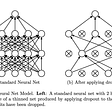## TrustSVD: Collaborative Filtering with Both the Explicit and Implicit Influence of User Trust and…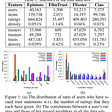## Decentralizing Machine Learning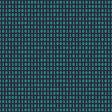## Generating Abstractive Summaries Using Google’s PEGASUS Model## 10 Key Talks from Spark Summit 2018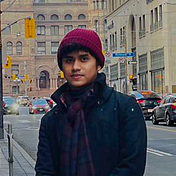noot noot.

## How to use facial landmarks obtained from dlib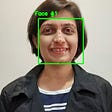## TensorFlow Image Processing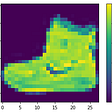## How to Detect Edges of an Image using Canny Edge Detection technique## MNIST image classification models from scratch